# Automated DETECT analysis using R

The post is organized as follows. First, we simulate a dataset using a multidimensional IRT model and compute the actual DETECT value using the correct item partitioning. Second, a brief description of the DETECT index is provided, and the DETECT value is calculated based on this definition based on the true item clustering for the simulated dataset. Then, we compute the same value using the original DETECT program by executing it through R. Finally, we conduct a simple simulation to demonstrate how to automate running DETECT to analyze many datasets and processing the DETECT output files in R.

Cengiz Zopluoglu (University of Oregon)
12-08-2020

If you ever study the multidimensional item response models, you will likely read about DETECT (Zhang & Stout, 1999) at some point as an alternative procedure for dimensionality assessment. DETECT, Dimensionality Evaluation to Enumerate Contributing Traits, is a conditional covariance-based nonparametric method to assess multidimensionality. The procedure was available as part of a commercial software package, and then it has been made public a while ago (DIMPACK software). The original software has user-friendly point-and-click interface, as shown below.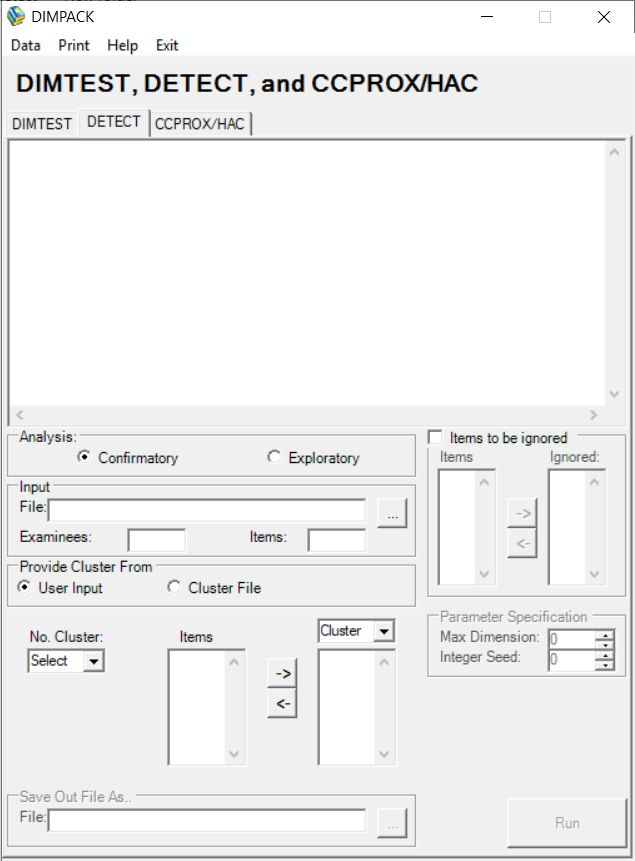Figure 1: A screenshot of user interface from DIMPACK

The user-friendly interface is not very helpful for simulation studies. So, one needs to find a way to automate running the DETECT procedure. In this post, I will demonstrate how to automate DETECT analysis using R. The post is organized as follows. First, we simulate a dataset using a multidimensional IRT model and compute the actual DETECT value using the correct item partitioning. Second, a brief description of the DETECT index is provided, and the DETECT value is calculated based on this definition based on the true item clustering for the simulated dataset. Then, we compute the same value using the original DETECT program by executing it through R. Finally, we conduct a simple simulation to demonstrate how to automate running DETECT to analyze many datasets and processing the DETECT output files.1

# A. Simulating Multidimensional Data

We are going to use a multidimensional compensatory 4-parameter logistic model in this demonstration. For simplicity, I present the equations for a two-dimensional model. This model’s details can be found in any IRT textbook, e.g., Reckase (2009), Bonifay (2020). In this model, the probability of a correct response is given by the following equation,

$P(Y=1 | \theta_1,\theta_2,a_1,a_2,d,g,u) = g + (u-g)\frac{1}{1 + e^{-(a_1\theta_1 + a_2 \theta_2+d)}},$

in which $$\theta_1$$ and $$\theta_2$$ are the person parameters for the two latent dimensions, $$a_1$$ and $$a_2$$ are the item discrimination parameters, $$g$$ is the item guessing parameter (lower bound), $$u$$ is the slipping parameter (upper bound), and $$d$$ is the intercept parameter.

Below, I adopted and slightly modified a table of item parameters from Reckase(2009) to generate data (Table 6.1).

ipar <- read.csv('data/ipar.csv')
ipar

     a1   a2     d    g    u
1  0.75 0.15  0.18 0.10 0.99
2  0.46 0.07 -0.19 0.05 0.98
3  0.86 0.40 -0.47 0.15 0.95
4  1.01 0.05 -0.43 0.10 0.97
5  0.55 0.15 -0.44 0.20 0.96
6  1.35 0.54 -0.58 0.20 0.94
7  1.38 0.47 -1.04 0.10 0.95
8  0.85 0.26  0.64 0.15 0.92
9  1.01 0.20  0.01 0.10 0.98
10 0.92 0.30  0.09 0.10 0.99
11 0.00 0.80  0.81 0.20 0.97
12 0.00 1.20 -0.19 0.10 0.96
13 0.05 0.71  0.45 0.15 0.95
14 0.01 2.14 -1.84 0.25 0.94
15 0.03 0.86  0.41 0.20 0.93
16 0.03 0.94 -0.30 0.10 0.92
17 0.03 1.36 -0.18 0.20 0.91
18 0.00 0.90  0.51 0.10 0.96
19 0.00 0.73  1.13 0.25 0.97
20 0.00 0.64  0.02 0.10 0.99

The below code generates dichotomous response data for 1000 hypothetical test-takers using the following steps:

1. Generate $$\theta_1$$ and $$\theta_2$$ from a multivariate distribution with μ=(0,0) and Σ= $$\begin{pmatrix} 1 & 0 \\ 0 & 1 \end{pmatrix}$$.

2. Compute the probability of correct response for each test-taker given the item and person parameters.

3. Compare the probability to a random number from a uniform distribution between 0 and 1. If the probability is higher than the random number, then assign 1. If the probability is smaller than the random number, then assign 0.

require(MASS)

resp <- matrix(nrow=1000,ncol=nrow(ipar))

set.seed(34)

th <- mvrnorm(1000,mu=c(0,0),Sigma=diag(2))

for(i in 1:nrow(ipar)){

a1 <- ipar[i,]$a1 a2 <- ipar[i,]$a2
d  <- ipar[i,]$d g <- ipar[i,]$g
u  <- ipar[i,]$u z <- a1*th[,1] + a2*th[,2] + d prob <- g + (u-g)*(exp(z)/(1+exp(z))) resp[,i] <- (runif(1000,0,1)<prob)*1 } colMeans(resp)    0.584 0.474 0.502 0.455 0.514 0.481 0.367 0.640 0.540 0.560 0.713  0.481 0.625 0.452 0.625 0.453 0.523 0.617 0.782 0.533 We can see the two strong dimensions reflected in the scree plot based on the tetrachoric correlation matrix’s eigenvalues. require(qgraph) corr <- cor_auto(resp) eigens <- eigen(corr)$values

eigens

  2.7873149 2.1473382 1.1808323 1.1481684 1.0895121 1.0119774
 1.0035682 0.9486724 0.8881906 0.8462036 0.8433042 0.7965349
 0.7465649 0.7237945 0.7178061 0.6903155 0.6475087 0.6125699
 0.5918369 0.5779865
plot(eigens)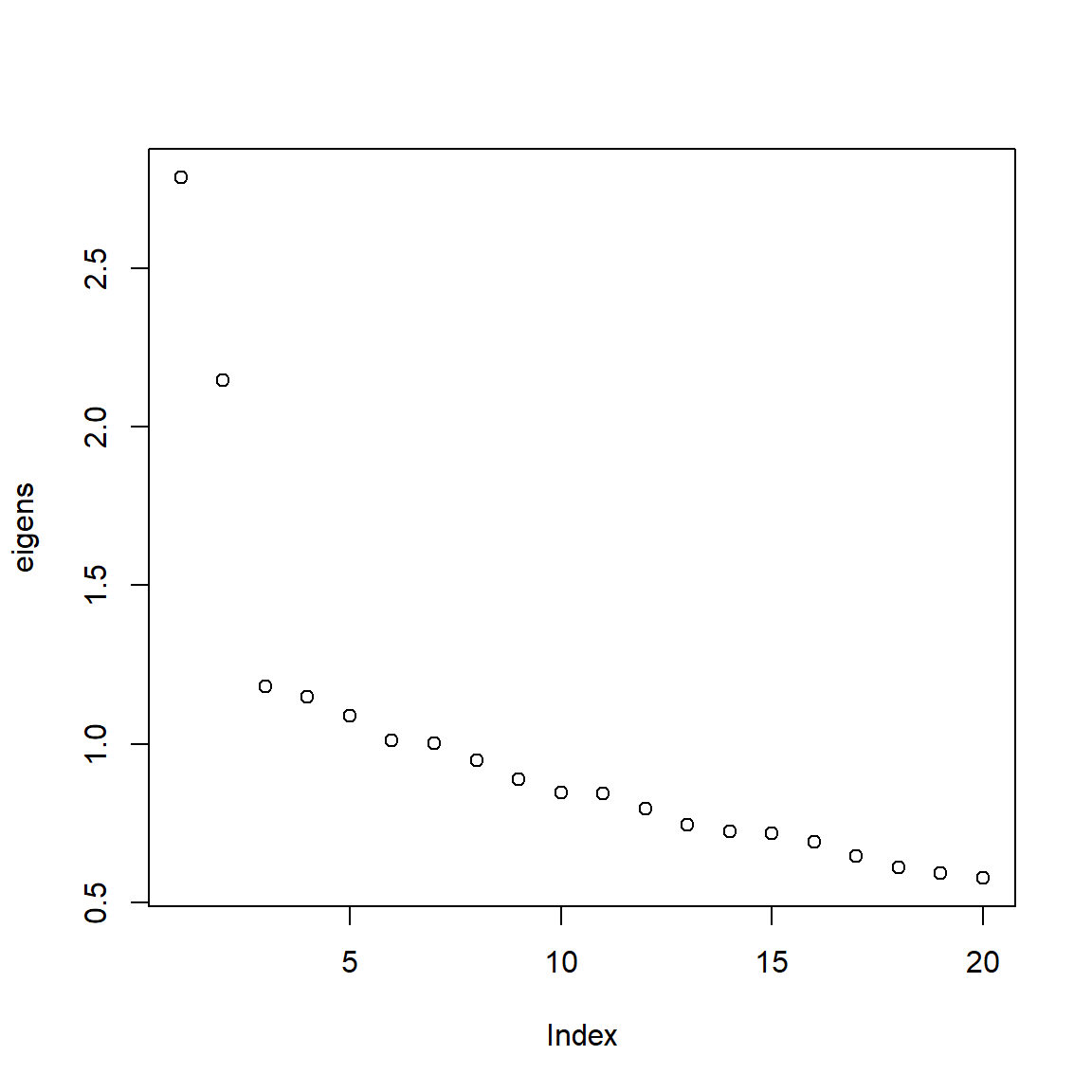Figure 2: Scree plot based on the eigenvalues calculated from tetrachoric correlation matrix

# B. DETECT Index

The DETECT index is based on the optimal partitioning of a set of items. The items with positive conditional covariances are grouped in the same clusters, and the items with negative conditional covariances are grouped in different clusters. Kim (1994) proposed the following quantity for a prespecified partitioning of a set of items (P):

$\mathrm{D}(\mathrm{P}) = \frac{2}{n(n-1)}\sum_{i=1}^{n-1}\sum_{j=i+1}^{n}\delta_{ij}C_{ij}(\mathbf{\theta_{\alpha}}),$ where $$n$$ is the number of items, $$\theta_{\alpha}$$ is a weighted composite of person parameters on multiple latent dimensions, $$C_{ij}(\mathbf{\theta_{\alpha}})$$ is the conditional covariance between the $$i$$th and $$j$$th items, $$\delta_{ij}$$ equals 1 if the $$i$$th and $$j$$th items are in the same cluster and –1 otherwise, and the summation is over all possible item pairs. We expect that a pair of items measuring the same dimension would have positive conditional covariance. In contrast, a pair of items measuring different dimensions would have a negative conditional covariance. $$D(P)$$ value drops if pairs of items with negative conditional covariances are assigned to the same cluster, or pairs of items with positive conditional covariances are assigned to different clusters. By contrast, the $$D(P)$$ value increases if pairs of items with negative conditional covariances are assigned to different clusters, or pairs of items with positive conditional covariances are assigned to the same cluster. Therefore, this quantity is designed to be maximized when you get the item partitioning right. Since we know the true item partitioning in the data simulated above, we can calculate this quantity in our simulated dataset based on the the true item partitioning.

When calculating the DETECT index for a given partitioning of items, the conditional covariance for each item pair must be computed, $$C_{ij}(\mathbf{\theta_{\alpha}})$$. A sum score is used as a proxy for the weighted composite of latent dimensions ($$\theta_{\alpha}$$) in these calculations, and two different estimates are obtained by using different types of sum scores. The first estimate uses all items in the test when computing a sum score. For an $$n$$-item test, the possible sum scores are from 0 to $$n$$. The whole sample is divided into $$n+1$$ score groups based on the sum scores, and then a weighted average is computed for covariance between item $$i$$ and item $$j$$,

$\hat{C}_{ij}^{1} = \sum_{k=0}^{n}\frac{J_k}{N}\hat{C}_{ij}(S=k),$

where $$J_k$$ is the number of people in the $$k$$th score group, $$N$$ is the total sample size, and $$S$$ is the sum score from all $$n$$ items.

The second estimate is very similar to the first one. The only difference is that a different sum score is computed for each individual based on the remaining $$n-2$$ items after excluding the $$i$$th and $$j$$th item when calculating $$C_{ij}(\mathbf{\theta_{\alpha}})$$, and this rest sum score is used for conditioning,

$\hat{C}_{ij}^{2} = \sum_{k=0}^{n-2}\frac{J_k}{N}\hat{C}_{ij}(S_{i,j}=k),$ where $$S_{i,j}$$ is the rest sum score for the remaining $$n-2$$ items after excluding item $$i$$ and item $$j$$.

$C_{ij}(\mathbf{\theta_{\alpha}}) = \frac{\hat{C}_{ij}^{1} + \hat{C}_{ij}^{2}}{2}$ Then, $$D(P)$$ is an aggregate measure of pairwise local dependence for the entire test.$$D(P)$$ should be equal to zero at the population level if the test is indeed unidimensional, and any departure from 0 is an indication of multidimensionality. $$D(P)$$ value is multiplied by 100 to make the interpretation easier.

Several cut-off values are given in the literature for different DETECT index levels (e.g., Stout, Nandakumar, Habing, 1996; Roussos & Ozbek, 2006), although it is questionable how useful these thresholds are in practice and whether or not they apply to all conditions. For instance, Stout et al. recommended the following classification based on the magnitude of D(P):

• Below 0.10 –> unidimensionality
• (0.10,0.50] –> weak multidimensionality
• (0.50,1.00] –> moderate multidimensionality
• (1.00,1.50] –> strong multidimensionality
• Above 1.50 –> very strong multidimensionality

Below, we compute the conditional covariance for 190 item pairs from the simulated dataset.

CCOV <- matrix(nrow=20,ncol=20)

for(i in 1:19){
for(j in (i+1):20){

ccov1 <- c()
S1    <- rowSums(resp)

for(k in 0:20){
if(length(which(S1==k))!=0){
sub = which(S1==k)
ccov1[k] = (cov(resp[sub,i],resp[sub,j]))*(length(sub)/nrow(resp))
}
}

ccov2 <- c()
S2    <- rowSums(resp[,-c(i,j)])

for(k in 0:18){
if(length(which(S2==k))!=0){
sub = which(S2==k)
ccov2[k] = (cov(resp[sub,i],resp[sub,j]))*(length(sub)/nrow(resp))
}
}

CCOV[i,j]=(sum(ccov1,na.rm=TRUE)+sum(ccov2,na.rm=TRUE))/2
}
}

data.frame(round(CCOV,3)) %>%
kbl() %>%
kable_classic(full_width = F)

X1 X2 X3 X4 X5 X6 X7 X8 X9 X10 X11 X12 X13 X14 X15 X16 X17 X18 X19 X20
0.004 0.015 0.006 0.006 -0.001 0.012 0.007 0.007 0.017 -0.009 -0.011 -0.010 -0.022 -0.028 -0.003 -0.016 -0.008 -0.013 -0.008
0.005 0.010 0.001 0.001 0.016 0.006 0.012 -0.001 -0.018 -0.006 -0.013 -0.005 -0.008 -0.020 -0.014 -0.010 -0.008 -0.014
0.017 -0.012 -0.006 0.006 0.016 0.011 0.013 -0.001 -0.012 -0.009 -0.014 -0.002 0.003 -0.014 -0.015 -0.010 -0.028
0.016 0.008 0.019 0.011 0.018 0.019 -0.009 -0.024 -0.023 -0.008 -0.012 -0.011 -0.028 -0.020 -0.012 -0.013
-0.011 0.002 0.013 0.005 0.007 -0.002 -0.025 -0.012 0.007 -0.009 -0.014 -0.019 -0.014 -0.014 -0.005
0.006 -0.001 0.002 -0.002 -0.007 -0.006 0.009 -0.001 -0.003 -0.026 0.007 -0.018 -0.006 -0.003
0.002 0.018 0.013 -0.016 -0.016 -0.003 -0.006 -0.021 -0.015 -0.006 -0.013 -0.006 -0.005
0.009 0.002 -0.009 -0.012 -0.016 -0.002 -0.005 -0.007 -0.018 -0.017 0.003 -0.011
0.021 -0.015 -0.013 -0.023 -0.020 -0.006 -0.015 -0.012 -0.014 -0.007 -0.006
-0.011 -0.005 -0.010 -0.019 -0.015 -0.014 -0.011 -0.012 -0.011 -0.003
0.003 0.013 -0.002 -0.002 0.011 0.009 0.001 0.004 0.015
0.007 0.018 0.014 0.018 0.016 0.013 0.000 0.008
0.007 0.008 0.001 0.017 0.017 0.005 0.002
0.005 0.014 0.018 0.016 0.001 -0.009
0.008 0.015 0.014 0.002 -0.006
0.000 0.012 0.009 0.001
0.007 0.006 0.005
0.004 0.001
0.010

Next, we need a similar matrix for $$\delta$$ values. The correct partitioning of items will be used to get the maximum DETECT value: Item 1 - Item 10 in Cluster 1 and Item 11-20 in Cluster 2. We assign 1 for the item pairs in the same cluster and -1 for the item pairs in different clusters.

cl1 <- 1:10
cl2 <- 11:20

pos.pairs <- data.frame(
rbind(
t(combn(cl1,2)),
t(combn(cl2,2)))
)
pos.pairs$val <- 1 neg.pairs <- expand.grid(X1=cl1,X2=cl2) neg.pairs$val <- -1

pairs <- rbind(pos.pairs,neg.pairs)

delta <- matrix(nrow=20,ncol=20)

for(i in 1:nrow(pairs)){
delta[pairs[i,1],pairs[i,2]] = pairs[i,3]
}

data.frame(delta) %>%
kbl() %>%
kable_classic(full_width = F)

X1 X2 X3 X4 X5 X6 X7 X8 X9 X10 X11 X12 X13 X14 X15 X16 X17 X18 X19 X20
1 1 1 1 1 1 1 1 1 -1 -1 -1 -1 -1 -1 -1 -1 -1 -1
1 1 1 1 1 1 1 1 -1 -1 -1 -1 -1 -1 -1 -1 -1 -1
1 1 1 1 1 1 1 -1 -1 -1 -1 -1 -1 -1 -1 -1 -1
1 1 1 1 1 1 -1 -1 -1 -1 -1 -1 -1 -1 -1 -1
1 1 1 1 1 -1 -1 -1 -1 -1 -1 -1 -1 -1 -1
1 1 1 1 -1 -1 -1 -1 -1 -1 -1 -1 -1 -1
1 1 1 -1 -1 -1 -1 -1 -1 -1 -1 -1 -1
1 1 -1 -1 -1 -1 -1 -1 -1 -1 -1 -1
1 -1 -1 -1 -1 -1 -1 -1 -1 -1 -1
-1 -1 -1 -1 -1 -1 -1 -1 -1 -1
1 1 1 1 1 1 1 1 1
1 1 1 1 1 1 1 1
1 1 1 1 1 1 1
1 1 1 1 1 1
1 1 1 1 1
1 1 1 1
1 1 1
1 1
1

To compute the DETECT index, we multiply the corresponding elements of these two matrices, take the average of this product, and multiply the average by 100.

mean(delta[upper.tri(delta)]*CCOV[upper.tri(CCOV)])*100

 0.9428365

# C. Analyzing the Simulated Dataset Using the DETECT program

When we know the true dimensional structure of data, it is straightforward to compute the DETECT index for a given dataset, and there is nothing that should excite us to do so. What is more exciting is to explore and try to reveal the dimensional structure when we don’t know the underlying structure. Imagine how many possible partitioning there exists for a given set of items. For instance, when you have a three-item test, there are five possible partitionings as follows:

• Each item loads on a different dimension: {(1),(2),(3)}
• Item 1 and 2 loads on one dimension, and Item 3 loads on another dimension: {(1,2),(3)}
• Item 1 and 3 loads on one dimension, and Item 2 loads on another dimension: {(1,3),(2)}.
• Item 2 and 3 loads on one dimension, and Item 2 loads on another dimension: {(2,3),(1)}.
• All items load on the same dimension, {(1,2,3)}

The total number of partitions for an n-item test is equal to a Bell number in mathematics and increases incredibly as the number of elements increases. For instance, the number of possible partitions reaches 115,975 for ten items. Thus, dimensionality analysis becomes an optimization problem through an exhaustive search for the optimal partitioning of items that give the maximum DETECT value. Kim (1994) originally proposed using some prior judgments with cluster analysis to begin. Then, (Zhang & Stout, 1999) developed a more sound solution by using a genetic algorithm to optimize the maximum DETECT value among all possible partitions of a set of items. In this optimization process, an informed choice of a partition is specified by the user (e.g., based on cluster analysis) to start. Then the genetic algorithm is used to find the optimum partitioning.

I wish I could have the skills to demonstrate how this algorithm works by walking you through some R code, but unfortunately, I don’t (maybe one day, there is always hope!). The original Fortran code for the software is available if you download the DIMPACK software. detect4.exe is a compiled Fortran code that requires a text file as an input to run the analysis for a given dataset.

The input text file for the detect4.exe program should look like this, and the extension of the file should be .in. In the next section, we will automate the creation of this input text file.

name of data file
C:/Users/cengiz/Desktop/Github/website/_posts/2020-12-04-detect/test/resp.dat
no.of items
20
no.of examinees
1000
mincell
2
mutations
4
max dimensions
12
dropflag
0
no.of items to drop from the analysis
0
items to be dropped
0
confimatory flag
0
crosflag
0
no.of examinees to set aside for cross validation
0
seed
99991
name of detect summary output file
C:/Users/cengiz/Desktop/Github/website/_posts/2020-12-04-detect/test/resp.out
cluster output flag
0
covariance output flag
0

The inputs in this file are:

• name of data file –> a path to the sample dataset to be analyzed

• no.of items –> number of items in the sample dataset

• no.of examinees –> number of examinees in the sample dataset

• mincell –> the minimum number of examinees required to be present in any one cell when calculating the conditional covariances

• mutations –> number of vectors mutated in the genetic algorithm. A fifth of the number of items recommended.

• max dimensions –> maximum number of dimensions to expand the search. Twelve is the maximum allowed.

• dropflag –> 0/1, 0 means no items dropped and the next 4 lines are ignored

• confimatory flag –> 0/1, 0 means exploratory DETECT analysis, 1 means confirmatory DETECT analysis. For confirmatory DETECT analysis, item clusters must be provided in a separate file.

• crosflag –> 0/1, 0 means no cross validation and next 2 lines ignored.

• no.of examinees to set aside for cross validation –> if crossflag is 1, then this is the number of examinees in the dataset to be used for cross validation

• seed –> seed number for random number generation. This number is used in the genetic algorithm random search mechanism and/or in randomly splitting the data if cross-validation is requested.

• name of detect summary output file –> the name for the output file to be written

• cluster output flag –> 0/1, 0 means no separate output file, 1 creates a separate file ‘cluster.out’ for item clusters

• covariance output flag –> 0/1 0 means no covariance output file, 1 creates ‘cov.out’ for conditional covariances calculated

Once this input file is created, we also need the data file to exist in the same directory. For DETECT analysis, I always prepare my datasets in fixed-width format such that each character corresponds to an item. You can use the following code to write the sample data file in fixed-width format.

  require(gdata)

write.fwf(resp,
here('_posts/2020-12-04-detect/test/resp.dat'),
width=rep(1,ncol(resp)),
na=' ',
sep='',
rownames=FALSE,colnames=FALSE)


Our data in fixed-width format should look like this.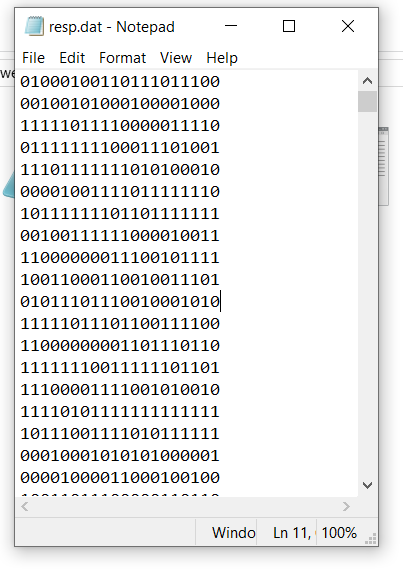Figure 3: A screenshot of fixed-width format data file

To run detect4.exe from R, you need to have these three files in the same folder.

1. The dataset as a text file in a fixed-width format (e.g., resp.dat)
2. The DETECT input file (detect.in)
3. detect4.exe file

Finally, we can invoke a system command to execute the analysis using the detect4.exe file for the given input file. In this case, I created a test folder, and all these files, including the detect4.exe, are located in .../_posts/2020-12-04-detect/test/.

    system(here('_posts/2020-12-04-detect/test/detect4.exe'))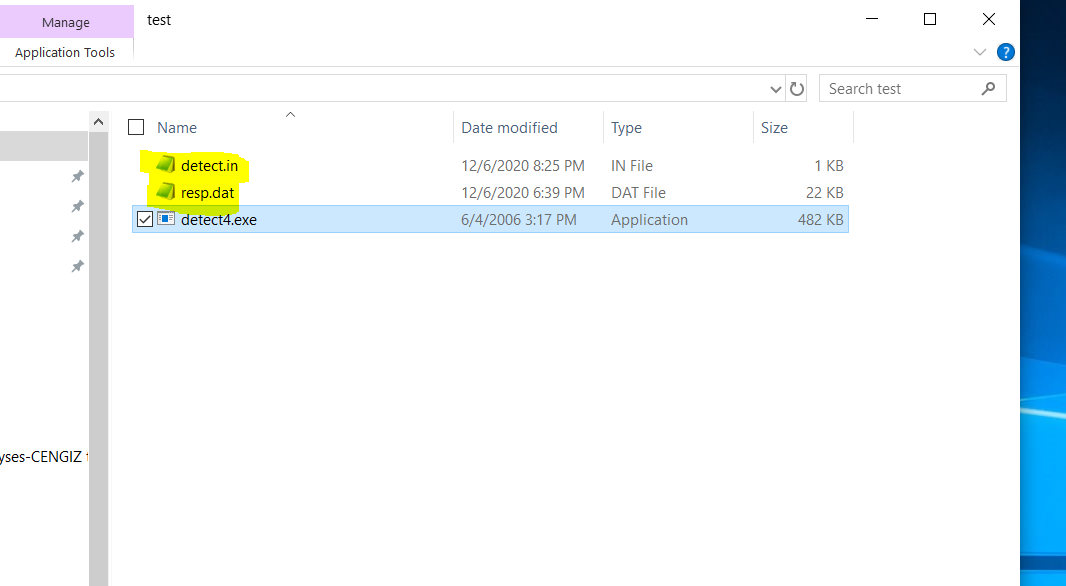Figure 4: Necessary files to run detect4.exe file

When the Fortran code is examined, it appears that detect4.exe specifically searches for a file labeled as detect.in in the same folder when it is invoked and always runs that file. Therefore, the input file in the folder must always be labeled as detect.in. This information is important for the next section when you analyze many datasets. You have to update the information in the detect.in file every time you analyze a different dataset. Once the analysis is done, the DETECT output file (resp.out) should be written in the same folder after the analysis.

-------------------------------------------------------
DETECT SUMMARY OUTPUT
-------------------------------------------------------

Data File Name: C:/Users/cengiz/Desktop/Github/website/_posts/2020-12-04-detect/test/resp.dat

Number of Items used:       20

Number of Items dropped:        0

Number of Examinees:     1000

Minimum Number of
Examinees per Cell:        2

Number of Vectors Mutated:        4

Maximum Number of Dimensions:       12

Randomization Seed:    99991

Minimum percentage of examinees
used after deleting cells
having less than  2 examinees:    99.70

Average percentage of examinees
used after deleting cells
having less than  2 examinees:    99.97

-------------------------------------------------------

NUMBER OF DIMENSIONS THAT MAXIMIZE DETECT:  3

Exploratory DETECT Statistics:

Maximum DETECT value:   0.9344
IDN index value:   0.9158
Ratio r:   0.9205

PARTITION WITH MAXIMUM DETECT VALUE:
....

For this particular simulated dataset, DETECT analysis found three dimensions. It got pretty close to the true structure. The only issue is that the analysis isolated Item 6 on its own from the other two clusters and incorrectly didn’t assign it to where it belongs. That is OK as there is no guarantee that the DETECT procedure will do a perfect job in revealing the underlying structure, and we can speculate that this is probably a sampling error in this case. The maximum DETECT value is reported as 0.9344. This number is slightly different than the maximum DETECT value of 0.9428 we calculated above for the correct partitioning. So, DETECT reports the value for what it thinks the optimal partitioning. Since DETECT couldn’t recover the true partitioning 100%, there is a slight deviation from the DETECT value we calculated for the true partitioning of items.

# D. Automating DETECT Runs for a Simulation Study

We have now a basic sense of what a DETECT input file and input dataset should look like and how we run it using the R system() command. This is all we need to automate many DETECT runs. In this post, I will consider studying the small sample performance of DETECT. For instance, how does the DETECT estimate’s sampling distribution look like when you have a small sample size (e.g., 250)? Is the DETECT estimate biased? How large is the standard error? The study by Roussos and Ozbek (2006) looked at this but for a sample size of 120,000. So, we will just replicate a small piece of it, but for the sample size of 250. Note that I am not aware of any large-sample theory developed for the DETECT statistic. We don’t have equations to calculate its standard error for given sample size, or we don’t necessarily know its distribution. A simulation-based approach seems to be the only way to do it.

We will need a few things to accomplish this. First, we would have to figure out the population level DETECT parameter for a given set of multidimensional item parameters. Roussos and Ozbek (2006) provide the equations to compute it, but they involve double integration. I can’t calculate it as they did in their paper using these equations (I am working on it!). Another way to get an idea about the population DETECT parameter for a given set of multidimensional item parameters is to simulate a dataset with a considerable sample size (e.g., 500,000) and then analyze it using the DETECT program. We can hope that the DETECT obtained from such a big dataset is pretty close to the population DETECT parameter given the set of item parameters used to generate the dataset. When I did that, I got an estimate of 0.899. The original DETECT program has a limit of 120,000 for sample size. So, I had to re-compile the original Fortran code by changing the limit from 120,000 to 500,000. So, let’s suppose that 0.899 is the population DETECT parameter for this particular set of multidimensional item parameters.

Second, we need a function to simulate data and write the simulated data in a proper format. Below is a function that takes sample size (N), item parameter matrix (ip), a path for a folder to store all the files generated (dir), and a file name (name).

sim.m4pl <- function(N,ip,dir,name){

#    N, sample size, integer

#   ip, item parameter matrix, five columns (a1, a2, d, g, and u)
#       each row represents an item

#  dir, path to a folder to store the files
#   e.g., C:/Users/cengiz/Desktop/test

# name, a name for the files to be used while writing the data into the folder

resp <- matrix(nrow=N,ncol=nrow(ip))

th <- mvrnorm(N,mu=c(0,0),Sigma=diag(2))

for(i in 1:nrow(ip)){

a1 <- ip[i,]$a1 a2 <- ip[i,]$a2
d  <- ip[i,]$d g <- ip[i,]$g
u  <- ip[i,]\$u

z    <- a1*th[,1] + a2*th[,2] + d
prob <- g + (u-g)*(exp(z)/(1+exp(z)))

resp[,i] <- (runif(N,0,1)<prob)*1
}

write.fwf(resp,
paste0(dir,'/',name,'.dat'),
width=rep(1,ncol(resp)),
na=' ',
sep='',
rownames=FALSE,colnames=FALSE)

return(resp=resp)

}


Once we have the function, we can simulate data using the multidimensional 4PL model with two dimensions for any given sample size and item parameter matrix. The following code will generate a dataset for 250 hypothetical individuals using the item parameter matrix we used before and export it to a text file (rep1.dat) in the specified folder using a fixed-width format.

d <- sim.m4pl(N=250,
ip=ipar,
dir= here('_posts/2020-12-04-detect/test'),
name='rep1')


Third, we need a function to update the detect.in file so that the program analyzes the right dataset every time we invoke the detect4.exe file. Below is a function that takes the generated dataset from the previous function (data), the name being used for saving the dataset, and the path for the same folder the dataset was saved (dir).

wrt.inp <- function(data,name,dir){

# data, item response data matrix with N rows and k items
# name, name for the text file the data is saved
# dir, path to the same folder the data is stored

ctl <- c("name of data file")
ctl <- rbind(ctl,paste0(dir,"/",name,".dat",sep=""))
ctl <- rbind(ctl,c("no.of items"))
ctl <- rbind(ctl,ncol(data))
ctl <- rbind(ctl,c("no.of examinees"))
ctl <- rbind(ctl,nrow(data))
ctl <- rbind(ctl,c("mincell"))
ctl <- rbind(ctl,2)
ctl <- rbind(ctl,c("mutations"))
ctl <- rbind(ctl,ncol(data)/5)
ctl <- rbind(ctl,c("max dimensions"))
ctl <- rbind(ctl,12)
ctl <- rbind(ctl,c("dropflag"))
ctl <- rbind(ctl,0)
ctl <- rbind(ctl,c("no.of items to drop from the analysis"))
ctl <- rbind(ctl,0)
ctl <- rbind(ctl,c("items to be dropped"))
ctl <- rbind(ctl,0)
ctl <- rbind(ctl,c("confimatory flag"))
ctl <- rbind(ctl,0)
ctl <- rbind(ctl,c("crosflag"))
ctl <- rbind(ctl,0)
ctl <- rbind(ctl,c("no.of examinees to set aside for cross validation"))
ctl <- rbind(ctl,0)
ctl <- rbind(ctl,c("seed"))
ctl <- rbind(ctl,99991 )
ctl <- rbind(ctl,c("name of detect summary output file"))
ctl <- rbind(ctl,paste0(dir,"/",name,".out",sep=""))
ctl <- rbind(ctl,c("cluster output flag"))
ctl <- rbind(ctl,0)
ctl <- rbind(ctl,c("covariance output flag"))
ctl <- rbind(ctl,0)
ctl <- noquote(ctl)
write(ctl,paste0(dir,"/detect.in",sep=""))
}


When we run the following code, it reproduces the detect.in file by overwriting the information for the following arguments according to the dataset and name provided:

• name of data file
• no.of items
• no.of examinees
• name of detect summary output file
wrt.inp(data=d,
name='rep1',
dir=here('_posts/2020-12-04-detect/test'))

name of data file
C:/Users/cengiz/Desktop/Github/website/_posts/2020-12-04-detect/test/rep1.dat
no.of items
20
no.of examinees
250
mincell
2
mutations
4
max dimensions
12
dropflag
0
no.of items to drop from the analysis
0
items to be dropped
0
confimatory flag
0
crosflag
0
no.of examinees to set aside for cross validation
0
seed
99991
name of detect summary output file
C:/Users/cengiz/Desktop/Github/website/_posts/2020-12-04-detect/test/rep1.out
cluster output flag
0
covariance output flag
0

We can now invoke a system command again to execute the analysis using detect4.exe for the dataset we just generated with a sample size of 250 using the same item parameters.

    system(here('_posts/2020-12-04-detect/test/detect4.exe'))

-------------------------------------------------------
DETECT SUMMARY OUTPUT
-------------------------------------------------------

Data File Name: C:/Users/cengiz/Desktop/Github/website/_posts/2020-12-04-detect/test/rep1.dat

Number of Items used:       20

Number of Items dropped:        0

Number of Examinees:      250

Minimum Number of
Examinees per Cell:        2

Number of Vectors Mutated:        4

Maximum Number of Dimensions:       12

Randomization Seed:    99991

Minimum percentage of examinees
used after deleting cells
having less than  2 examinees:    98.80

Average percentage of examinees
used after deleting cells
having less than  2 examinees:    99.50

-------------------------------------------------------

NUMBER OF DIMENSIONS THAT MAXIMIZE DETECT:  4

Exploratory DETECT Statistics:

Maximum DETECT value:   0.7276
IDN index value:   0.6842
Ratio r:   0.5866

PARTITION WITH MAXIMUM DETECT VALUE:
....

This outcome is only for one replication. What would happen if we repeated the same process 1000 times? We can now generate 1000 datasets, update the detect.in for each one of them, and then invoke a system command again to execute the analysis. With all the machinery we described above, it is straightforward through a for loop. Note that this may take a long time depending on your computer configurations. So, you have to be patient. Alternatively, we may parallelize this process to take advantage of multiple cores in most computers. Suppose your computer has 16 cores. We can utilize 10 of those cores and divide this for loop such that it runs 100 replications in each core simultaneously. Parallelizing would significantly reduce the computational time but requires some more coding experience. So, I will leave it to another post.

for(i in 1:1000){

filename <- paste0('rep',i)

d <- sim.m4pl(N=250,
ip=ipar,
dir= here('_posts/2020-12-04-detect/test'),
name=filename)

wrt.inp(data=d,
name=filename,
dir=here('_posts/2020-12-04-detect/test'))

system(here('_posts/2020-12-04-detect/test/detect4.exe'))

print(i)
}


Once this process is done, you should have a folder with thousands of files, and it should look like this. Each file with an extension .out is the DETECT output file for the corresponding dataset.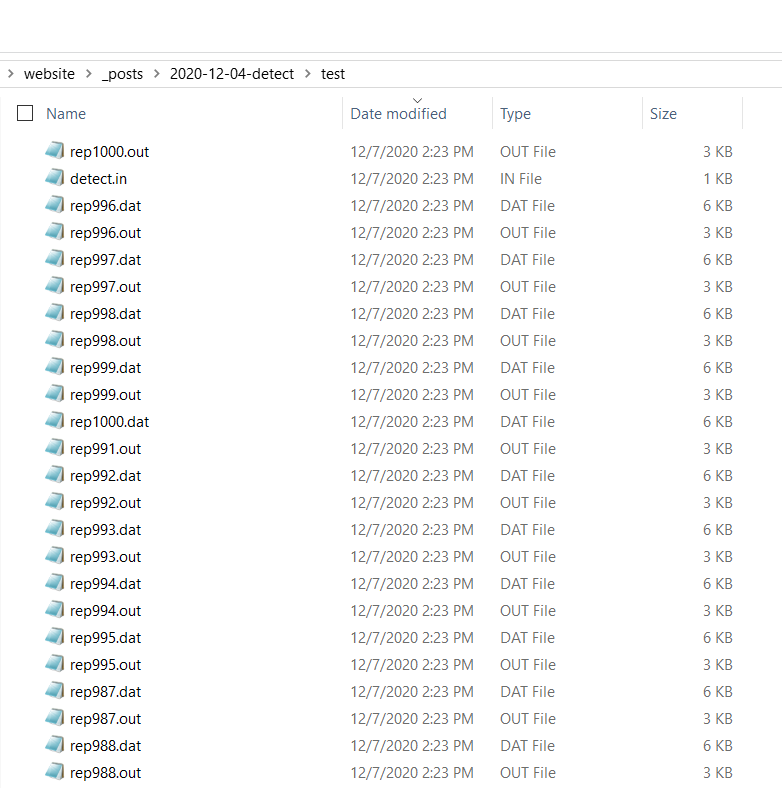Figure 5: Simulated datasets and corresponding DETECT output files

We are getting closer to the finish line. We still need to do some coding to extract all the information we need from these output files. Something that makes the extraction easier is that the output files are standardized and have almost the same structure. Here is how I approach it. First, I read an output file as a vector using the scan() function.

output <- scan(here('_posts/2020-12-04-detect/test/rep1.out'),what=c('raw'))

output


 “——————————————————-”
 “DETECT”
 “SUMMARY”
 “OUTPUT”
 “——————————————————-”
 “Data”
 “File”
 “Name:”
 "C:/Users/cengiz/Desktop/Github/website/_posts/2020-12-04-detect/test/rep1.dat"  “Number”
 “of”
 “Items”
 “used:”
 “20”
 “Number”
 “of”
 “Items”
 “dropped:”
 “0”
 “Number”
 “of”
 “Examinees:”
 “250”
 “Minimum”
 “Number”
 “of”
 “Examinees”
 “per”
 “Cell:”
 “2”
 “Number”
 “of”
 “Vectors”
 “Mutated:”
 “4”
 “Maximum”
 “Number”
 “of”
 “Dimensions:”
 “12”
 “Randomization”
 “Seed:”
 “99991”
 “Minimum”
 “percentage”
 “of”
 “examinees”
 “used”
 “after”
 “deleting”
 “cells”
 “having”
 “less”
 “than”
 “2”
 “examinees:”
 “98.80”
 “Average”
 “percentage”
 “of”
 “examinees”
 “used”
 “after”
 “deleting”
 “cells”
 “having”
 “less”
 “than”
 “2”
 “examinees:”
 “99.50”
 “——————————————————-”
 “NUMBER”
 “OF”
 “DIMENSIONS”
 “THAT”
 “MAXIMIZE”
 “DETECT:”
 “4”
 “Exploratory”
 “DETECT”
 “Statistics:”
 “Maximum”
 “DETECT”
 “value:”
 “0.7276”
 “IDN”
 “index”
 “value:”
 “0.6842”
 “Ratio”
 “r:”
 “0.5866”
 “PARTITION”
 “WITH”
 “MAXIMUM”
 “DETECT”
 “VALUE:”
 “1”
 “2”
 “2”
 “2”
 “1”
 “1”
 “2”
 “1”
 “2”
 “2”
 “3”
 “3”
 “3”
 “4”
 “3”
 “3”
 “3”
 “3”
 “4”
 “3”
 “CLUSTER”
 “MEMBERSHIPS:”
 “———–CLUSTER”
 “1————-”
 “1”
 “5”
 “6”
 “8”
 “———–CLUSTER”
 “2————-”
 “2”
 “3”
 “4”
 “7”
 “9”
 “10”
 “———–CLUSTER”
 “3————-”
 “11”
 “12”
 “13”
 “15”
 “16”
 “17”
 “18”
 “20”
 “———–CLUSTER”
 “4————-”
 “14”
 “19”
 “———————————-”
 “Covariance”
 “Sign”
 “Pattern”
 “Matrix:”
 “d++—-++–+-+—-+-”
 “+d+-++-+-+—+—++-”
 “++d++–+-+–+-+—++”
 “–+d-+++—+-+–+—”
 “-++-d+-+++—–++—”
 “-+-++d+-+—–+–+-+”
 “—+-+d-++-+——-+”
 “+++++–d++-++-+-+—”
 “+—++++d–+—+-+-+”
 “-++-+-++-d-+——++”
 “———-d+++++-++-”
 “+–+–+++++d++-+++-+”
 “–+—-+–++d++-++++”
 “++-+——+++d++++–”
 “–+–+-+–+-++d+–+-”
 “—-+—+-++-++d++–”
 “—++–+—+++-+d+–”
 “-+—+–+-++++-++d–”
 “+++——++-+-+—d+”
 “–+–++-++-++—–+d”
 “No”
 “cross”
 “validation”
 “for”
 “this”
 “DETECT”
 “run”

This vector will look alike for almost all datasets being analyzed. I am interested in three pieces of information in this output.

1. DETECT estimate
2. Number of dimensions
3. The proportion of item pairs correctly assigned to clusters

For instance, the DETECT estimate is reported as element 86 for this particular output file; however, its position may slightly change from file to file. We need a unique text that appears in the output file to help identify its position. For instance, the word Statistics: only appears once in this output file, and the DETECT estimate is reported four elements after Statistics: no matter what dataset being analyzed. I can use Statistics: as a reference point to extract the DETECT estimate like the following.

 as.numeric(output[which(output=='Statistics:')+4])


 0.7276

I can use a similar approach for extracting the estimated number of dimensions. The word MAXIMIZE only appears once in this output file, and the number of estimated dimensions is reported two elements after MAXIMIZE.

 as.numeric(output[which(output=='MAXIMIZE')+2])


 4

Item partitioning is a little trickier but still possible. The word PARTITION only appears once in this output file, and the first element of partitioning information is reported five elements after PARTITION. The word MEMBERSHIPS: only appears once in this output file, and the last element of partitioning information is reported two elements before MEMBERSHIPS:.

 first <- which(output=='PARTITION')+5
last  <- which(output=='MEMBERSHIPS:')-2

as.numeric(output[first:last])


 1 2 2 2 1 1 2 1 2 2 3 3 3 4 3 3 3 3 4 3

This vector displays the assigned cluster for each item. We can compare it to true partitioning. This may be done in many ways. Below is my approach. I create a vector for true cluster assignments (true.cl), and I also store the estimated cluster assignments as a vector (est.cl). Then, I create two 20 by 20 matrix, one for true assignments (pop.cl) and one for estimated assignments (sam.cl). I run a double for loop to check each item pair. If two items are in the same cluster, I assign 1 and 0 otherwise for both the true and estimated cluster assignments. In the end, I compare two matrices. The item pairs with matching code (either 1 or 0) are the correct assignments based on the analysis. We can divide the total number of correct assignments by 190 (all possible pairs for 20 items) to find the proportion of item pairs correctly assigned by the analysis. For this particular dataset, 78.9% of all possible item pairs are correctly assigned by DETECT.

  est.cl <- as.numeric(output[first:last])

true.cl <- c(rep(1,10),rep(2,10))

pop.cl <- matrix(nrow=20,ncol=20)
sam.cl <- matrix(nrow=20,ncol=20)

for(i in 1:19){
for(j in (i+1):20){
pop.cl[i,j] = ifelse(true.cl[i]==true.cl[j],1,0)
sam.cl[i,j] = ifelse(est.cl[i]==est.cl[j],1,0)
}
}

data.frame(pop.cl) %>%
kbl() %>%
kable_classic(full_width = F)

X1 X2 X3 X4 X5 X6 X7 X8 X9 X10 X11 X12 X13 X14 X15 X16 X17 X18 X19 X20
1 1 1 1 1 1 1 1 1 0 0 0 0 0 0 0 0 0 0
1 1 1 1 1 1 1 1 0 0 0 0 0 0 0 0 0 0
1 1 1 1 1 1 1 0 0 0 0 0 0 0 0 0 0
1 1 1 1 1 1 0 0 0 0 0 0 0 0 0 0
1 1 1 1 1 0 0 0 0 0 0 0 0 0 0
1 1 1 1 0 0 0 0 0 0 0 0 0 0
1 1 1 0 0 0 0 0 0 0 0 0 0
1 1 0 0 0 0 0 0 0 0 0 0
1 0 0 0 0 0 0 0 0 0 0
0 0 0 0 0 0 0 0 0 0
1 1 1 1 1 1 1 1 1
1 1 1 1 1 1 1 1
1 1 1 1 1 1 1
1 1 1 1 1 1
1 1 1 1 1
1 1 1 1
1 1 1
1 1
1
data.frame(sam.cl) %>%
kbl() %>%
kable_classic(full_width = F)

X1 X2 X3 X4 X5 X6 X7 X8 X9 X10 X11 X12 X13 X14 X15 X16 X17 X18 X19 X20
0 0 0 1 1 0 1 0 0 0 0 0 0 0 0 0 0 0 0
1 1 0 0 1 0 1 1 0 0 0 0 0 0 0 0 0 0
1 0 0 1 0 1 1 0 0 0 0 0 0 0 0 0 0
0 0 1 0 1 1 0 0 0 0 0 0 0 0 0 0
1 0 1 0 0 0 0 0 0 0 0 0 0 0 0
0 1 0 0 0 0 0 0 0 0 0 0 0 0
0 1 1 0 0 0 0 0 0 0 0 0 0
0 0 0 0 0 0 0 0 0 0 0 0
1 0 0 0 0 0 0 0 0 0 0
0 0 0 0 0 0 0 0 0 0
1 1 0 1 1 1 1 0 1
1 0 1 1 1 1 0 1
0 1 1 1 1 0 1
0 0 0 0 1 0
1 1 1 0 1
1 1 0 1
1 0 1
0 1
0
  nacc = sum(pop.cl[upper.tri(pop.cl)]==sam.cl[upper.tri(sam.cl)])

nacc/190


 0.7894737

We can now run this for each output file and store the information in different objects for further analysis.

detect   <- c()  # vector to store DETECT estimates from 1000 runs
ndim     <- c()  # vector to store estimated number of dimensions from 1000 runs
accuracy <- c()  # vector to accuracy of cluster assignments

for(r in 1:1000){

filename    <- paste0('rep',r,'.out')

output      <- scan(here('_posts/2020-12-04-detect/test/',filename),what=c('raw'))

detect[r]   <-  as.numeric(output[which(output=='Statistics:')+4])

ndim[r]     <-  as.numeric(output[which(output=='MAXIMIZE')+2])

est.cl  <- as.numeric(output[first:last])

true.cl <- c(rep(1,10),rep(2,10))

pop.cl <- matrix(nrow=20,ncol=20)
sam.cl <- matrix(nrow=20,ncol=20)

for(i in 1:19){
for(j in (i+1):20){
pop.cl[i,j] = ifelse(true.cl[i]==true.cl[j],1,0)
sam.cl[i,j] = ifelse(est.cl[i]==est.cl[j],1,0)
}
}

nacc = sum(pop.cl[upper.tri(pop.cl)]==sam.cl[upper.tri(sam.cl)])

accuracy[r] <- nacc/190

}


Let’s check the distribution of DETECT estimates for 1000 datasets with a sample size of 250. The mean is 0.899, which is identical to the population value I approximated with a sample size of 500,000. We also get the standard deviation of 0.13, which may be used to approximate the DETECT estimate’s standard error when the sample size is 250 for this particular set of multidimensional item parameters.

hist(detect,prob=TRUE,col='white')
lines(density(detect))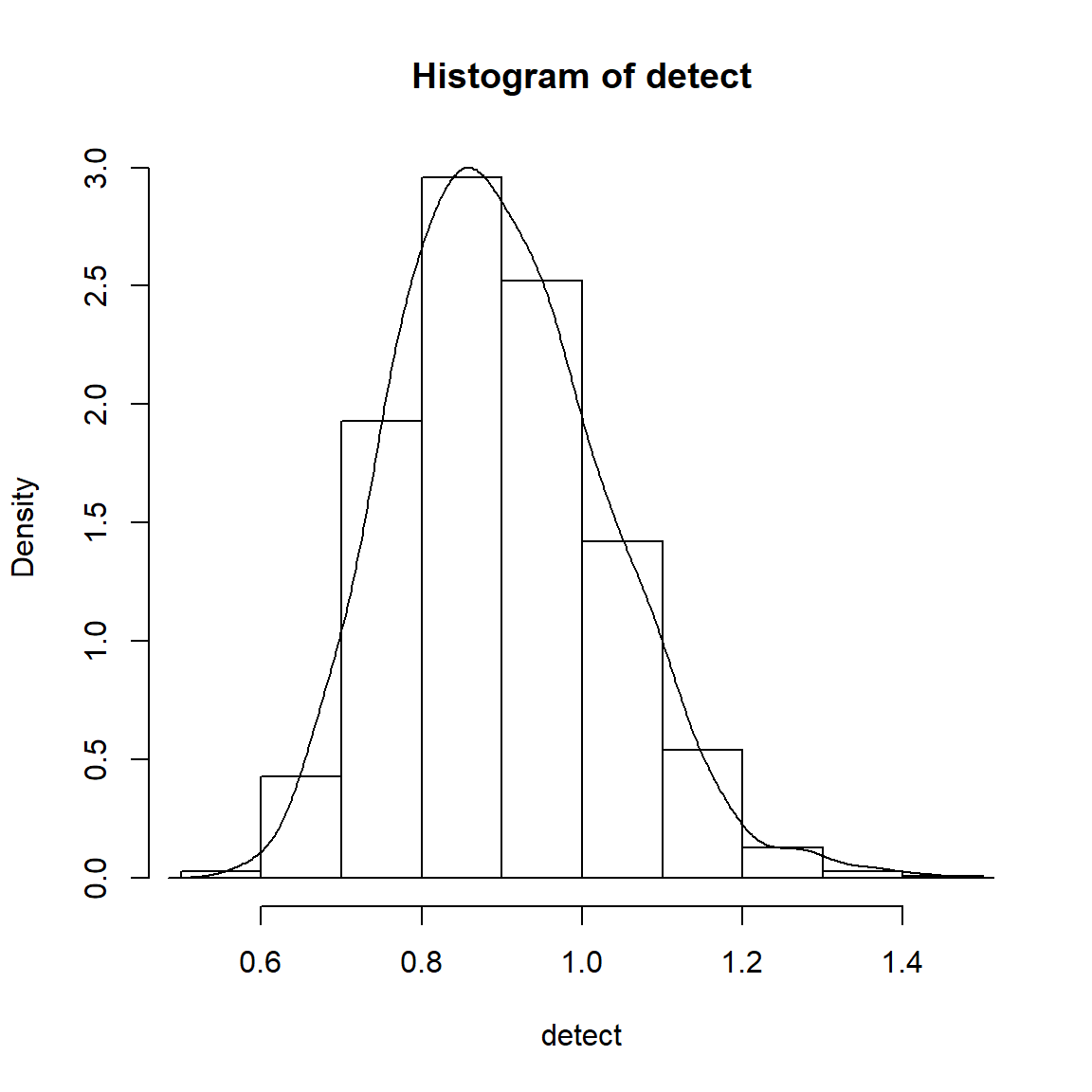Figure 6: Sampling distribution of DETECT estimate (N=250) for a particular set of multidimensional item parameters

mean(detect)

 0.8994012
sd(detect)

 0.1311087

How about the number of estimated dimensions? It appears that DETECT has found 2 dimensions for 14% of our replications. It may be concerning that DETECT found either 3 or 4 dimensions for the majority of replications. These replications with 3 or 4 dimensions probably include only one or two items in the extra dimensions and may practically be considered as two dimensions. However, one must write more code to dive into this issue and explore it further. I am not doing it here, as I start feeling that this post will never end.

table(ndim)/1000

ndim
2     3     4     5     6
0.141 0.533 0.288 0.036 0.002 

Finally, we can check the distribution of cluster assignment accuracy. On average, DETECT assigned 84% of all item pairs to the correct clusters.

hist(accuracy,prob=TRUE,col='white')
lines(density(accuracy))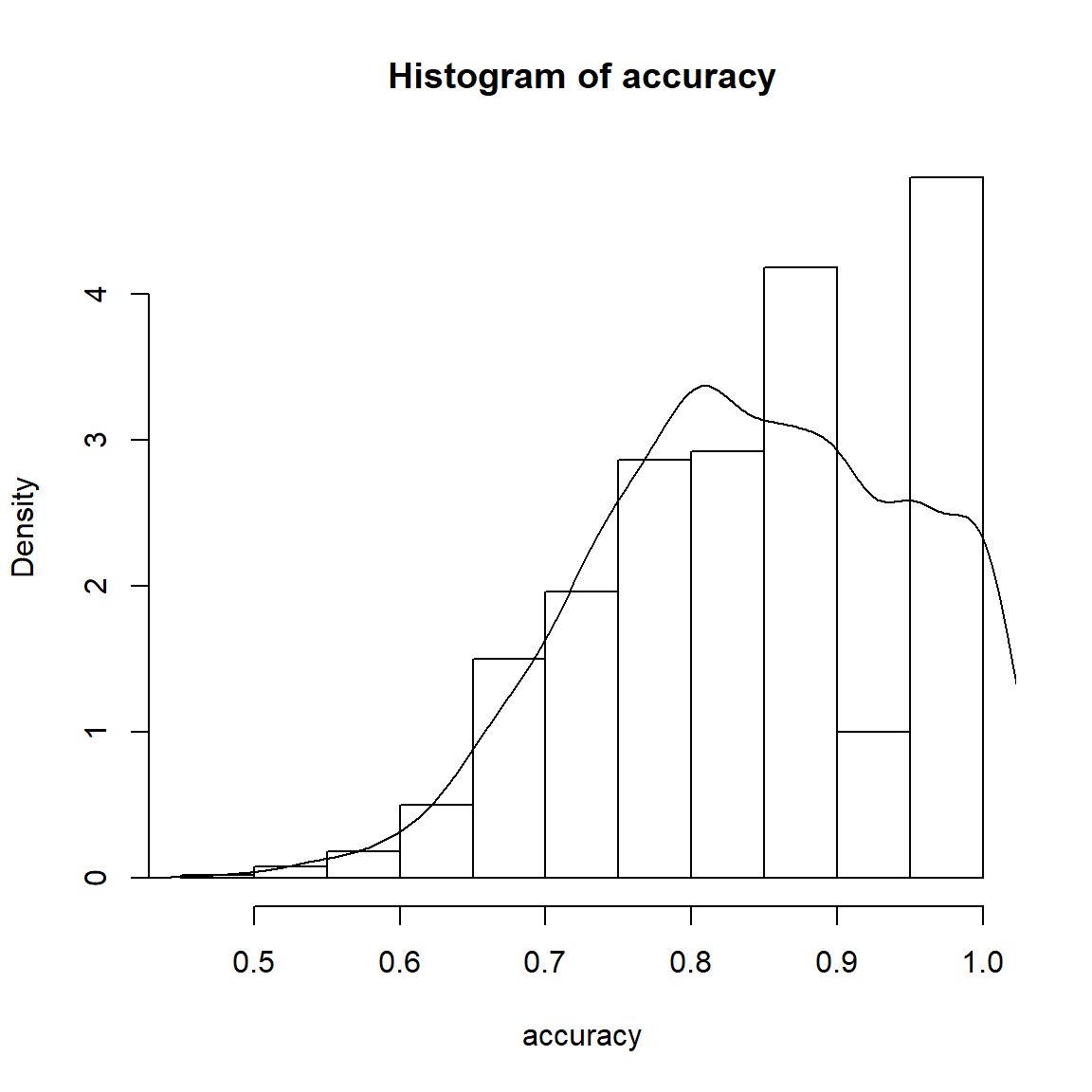Figure 7: Sampling distribution of cluster assignment accuracy for a particular set of multidimensional item parameters

mean(accuracy)

 0.8404421
sd(accuracy)

 0.1059856

I can’t believe you have read the whole thing! I hope it was worth your time. Thank you.

1. The sirt package in R has two functions, expl.detect() and conf.detect(), for running the DETECT procedure. In a recent attempt, I could not reproduce some of the analysis I conducted in the original DETECT software using these functions. I had some concerns about what these functions produce, particularly the function for the exploratory DETECT procedure. You can find more detail about these concerns at this link. ↩︎

### Citation

Zopluoglu (2020, Dec. 8). Cengiz Zopluoglu: Automated DETECT analysis using R. Retrieved from https://github.com/czopluoglu/website/tree/master/docs/posts/2020-12-04-detect/
@misc{zopluoglu2020automated,
}